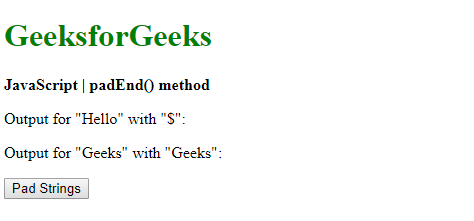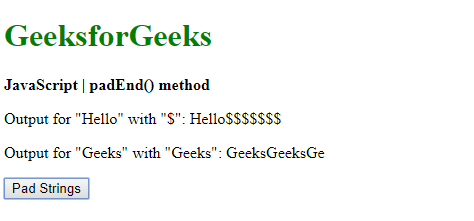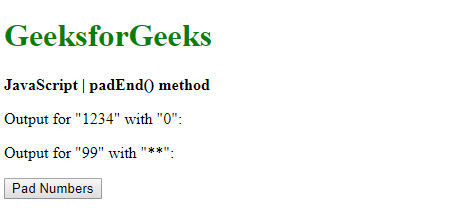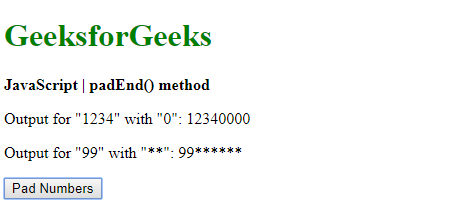The padEnd() method in JavaScript is used to pad a string with another string until it reaches the given length. The padding is applied from the right end of the string.

Syntax:

`string.padEnd( targetLength, padString )`

Parameters: This method accepts two parameters as mentioned above and described below:

• targetLength: It is the length of the final string once the original string has been padded. If the value is less than the original string length, then the original string is returned.
• padString: It is the string that is to be padded with the original string. If this value is too long to be within the targetLength, it is truncated.

Return Value: It returns the final string that is padded with the given string of the given length.

Example 1: This example uses padEnd() method to pad strings into another string.

 ` ` `<``html``> ` ` `  `<``head``> ` `    ``<``title``> ` `        ``JavaScript | padEnd() method ` `    `` ` ` ` ` `  `<``body``> ` `    ``<``h1` `style``=``"color: green"``> ` `        ``GeeksforGeeks ` `    `` ` `     `  `    ``<``b``> ` `        ``JavaScript | padEnd() method ` `    `` ` `     `  `    ``<``p``> ` `        ``Output for "Hello" with "\$":  ` `        ``<``span` `class``=``"output"``> ` `    `` ` `     `  `    ``<``p``> ` `        ``Output for "Geeks" with "Geeks":  ` `        ``<``span` `class``=``"output2"``> ` `    `` ` `     `  `    ``<``button` `onclick``=``"padStrings()"``> ` `        ``Pad Strings ` `    `` ` `     `  `    ``<``script` `type``=``"text/javascript"``> ` `         `  `        ``function padStrings() { ` `            ``exString = "Hello"; ` `            ``exString2 = "Geeks"; ` `         `  `            ``output =  ` `                ``exString.padEnd(12, "\$"); ` `            ``output2 =  ` `                ``exString2.padEnd(12, "Geeks"); ` `         `  `            ``document.querySelector('.output').textContent ` `                    ``= output; ` `             `  `            ``document.querySelector('.output2').textContent ` `                    ``= output2; ` `        ``} ` `    `` ` ` ` ` `  ` `

Output:

• Before clicking the button:• After clicking the button:Example 2: This example uses padEnd() method to pad numbers into the another number.

 ` ` `<``html``> ` ` `  `<``head``> ` `    ``<``title``> ` `        ``JavaScript | padEnd() method ` `    `` ` ` ` ` `  `<``body``> ` `    ``<``h1` `style``=``"color: green"``> ` `        ``GeeksforGeeks ` `    `` ` `     `  `    ``<``b``> ` `        ``JavaScript | padEnd() method ` `    `` ` `     `  `    ``<``p``> ` `        ``Output for "1234" with "0":  ` `        ``<``span` `class``=``"output"``> ` `    `` ` `     `  `    ``<``p``> ` `        ``Output for "99" with "**":  ` `        ``<``span` `class``=``"output2"``> ` `    `` ` `     `  `    ``<``button` `onclick``=``"padNumbers()"``> ` `        ``Pad Numbers ` `    `` ` `     `  `    ``<``script` `type``=``"text/javascript"``> ` `        ``function padNumbers() { ` `            ``exNumber = 1234; ` `            ``exNumber2 = 99; ` `         `  `            ``output =  ` `                ``String(exNumber).padEnd(8, "0"); ` `                 `  `            ``output2 =  ` `                ``String(exNumber2).padEnd(8, "**"); ` `                 `  `         `  `            ``document.querySelector('.output').textContent ` `                    ``= output; ` `             `  `            ``document.querySelector('.output2').textContent ` `                    ``= output2; ` `        ``} ` `    `` ` ` ` ` `  ` `

Output:

• Before clicking the button:• After clicking the button:Supported Browsers: The browser supported by padEnd() method are listed below:

• Firefox 48
• Edge 15
• Safari 10
• Opera 44My Personal Notes arrow_drop_upCheck out this Author's contributed articles.

If you like GeeksforGeeks and would like to contribute, you can also write an article using contribute.geeksforgeeks.org or mail your article to contribute@geeksforgeeks.org. See your article appearing on the GeeksforGeeks main page and help other Geeks.

Please Improve this article if you find anything incorrect by clicking on the "Improve Article" button below.